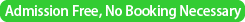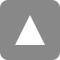﻿ 第30回統計的機械学習セミナー / The 30th Statistical Machine Learning Seminar | 統計数理研究所

# 第30回統計的機械学習セミナー / The 30th Statistical Machine Learning Seminar

Date&Time
2016年4月8日（金）15:00 - 16:00
/ 8 April, 2016 (Fri) 15:00 - 16:00Place

/ Seminar Room 5 (3F) @ The Institute of Statistical MathematicsSpeaker
Luigi Malago (Assistant Professor, Shinshu University)
Title
Second-order information geometry of Hessian manifolds
Abstract

In this talk we explore the second-order geometry of an exponential family, and more in general of Hessian manifolds, with the purpose of applying second-order methods to the optimization of functions defined over statistical models. The optimization of a cost function over a statistical model is a very general problem in stochastic optimization and machine learning: indeed it appears in many different contexts, such as in maximum-likelihood estimation, reinforcement learning, training of neural networks, Bayesian optimization, variational inference and many others. Second-order algorithms, such as the Newton method, are very popular techniques in optimization, which exploit second-order information of the function to be optimized, for instance by the evaluation of the Hessian. Second-order methods show super-linear convergence properties and, compared to first-order techniques, they are better suited for the optimization of ill-conditioned functions. However they require a higher computational cost, which may prevent their use in the high-dimensional setting. In Information Geometry, statistical models are represented as manifolds of probability distributions, where the Fisher information metric plays the role of metric tensor. As a consequence, the optimization over statistical models belongs to the more general field of Riemannian manifold optimization, so that first and second-order manifold optimization algorithms can be directly applied. Indeed, not surprisingly, the natural gradient corresponds to the Riemannian gradient evaluated with respect to the Fisher information metric. When we move to the second-order geometry of a differentiable manifold, the notion of covariant derivative is required for the parallel transport between tangent spaces, in particular to compute directional derivatives of vector fields over a manifold. However, an important result in Information Geometry affirms that exponential families, and more in general Hessian manifolds, have a dually-flat nature, which implies the existence of at least two other relevant geometries for statistical models: the mixture and the exponential geometries.

Differently from the Riemannian geometry, the exponential and mixture geometries are independent from the notion of metric, and they are defined by two dual affine connections, the mixture and the affine connections. The dual connections, which are equivalent specified by the dual covariant derivatives, allow to define dual parallel transports, dual geodetics, and ultimately the exponential and mixture Hessians. What is specific of Hessian manifolds, is that the combination of dual Hessians and geodetics allows to define alternative second-order Taylor approximations of a function, which do not require the computation of the Riemannian Hessian and geodetic. It follows that, compared to Riemannian manifolds, Hessian manifolds have a richer geometry that can be exploited in the design of more sophistical second-order optimization algorithms.